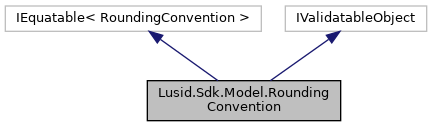LUSID C# SDK
Lusid.Sdk.Model.RoundingConvention Class Reference

Certain bonds will follow certain rounding conventions. For example, Thai government bonds will round accrued interest and cashflow values 2dp, whereas for French government bonds, the rounding is to 7dp. More...

Inheritance diagram for Lusid.Sdk.Model.RoundingConvention:[legend]

## Public Member Functions

RoundingConvention (decimal faceValue=default(decimal), int precision=default(int), string roundingTarget=default(string), string roundingType=default(string))
Initializes a new instance of the RoundingConvention class. More...

override string ToString ()
Returns the string presentation of the object More...

virtual string ToJson ()
Returns the JSON string presentation of the object More...

override bool Equals (object input)
Returns true if objects are equal More...

bool Equals (RoundingConvention input)
Returns true if RoundingConvention instances are equal More...

override int GetHashCode ()
Gets the hash code More...

## Properties

decimal FaceValue` [get, set]`
The face value to round against. The number to be rounded is scaled to this face value before being rounded, and then re-scaled to the holding amount. For example if rounding an accrued interest value using a FaceValue of 1,000, but 10,000 units are held, then the initial calculated value would be divided by 10,000, then multiplied by 1,000 and rounded per the convention. The result of this would then be divided by 1,000 and multiplied by 10,000 to get the final value. More...

int Precision` [get, set]`
The precision of the rounding. The decimal places to which the rounding takes place. More...

string RoundingTarget` [get, set]`
The target of the rounding convention. Accepted values are &#39;AccruedInterest&#39;, &#39;Cashflows&#39;, or &#39;All&#39; Supported string (enumeration) values are: [All, AccruedInterest, Cashflows]. More...

string RoundingType` [get, set]`
The type of rounding. e.g. Round Up, Round Down Supported string (enumeration) values are: [Down, Up, Floor, Ceiling, Nearest]. More...

## Detailed Description

Certain bonds will follow certain rounding conventions. For example, Thai government bonds will round accrued interest and cashflow values 2dp, whereas for French government bonds, the rounding is to 7dp.

## ◆ RoundingConvention()

 Lusid.Sdk.Model.RoundingConvention.RoundingConvention ( decimal faceValue = `default(decimal)`, int precision = `default(int)`, string roundingTarget = `default(string)`, string roundingType = `default(string)` )
inline

Initializes a new instance of the RoundingConvention class.

Parameters
 faceValue The face value to round against. The number to be rounded is scaled to this face value before being rounded, and then re-scaled to the holding amount. For example if rounding an accrued interest value using a FaceValue of 1,000, but 10,000 units are held, then the initial calculated value would be divided by 10,000, then multiplied by 1,000 and rounded per the convention. The result of this would then be divided by 1,000 and multiplied by 10,000 to get the final value.. precision The precision of the rounding. The decimal places to which the rounding takes place.. roundingTarget The target of the rounding convention. Accepted values are 'AccruedInterest', 'Cashflows', or 'All' Supported string (enumeration) values are: [All, AccruedInterest, Cashflows].. roundingType The type of rounding. e.g. Round Up, Round Down Supported string (enumeration) values are: [Down, Up, Floor, Ceiling, Nearest]..

## ◆ Equals() [1/2]

 override bool Lusid.Sdk.Model.RoundingConvention.Equals ( object input )
inline

Returns true if objects are equal

Parameters
 input Object to be compared
Returns
Boolean

## ◆ Equals() [2/2]

 bool Lusid.Sdk.Model.RoundingConvention.Equals ( RoundingConvention input )
inline

Returns true if RoundingConvention instances are equal

Parameters
 input Instance of RoundingConvention to be compared
Returns
Boolean

## ◆ GetHashCode()

 override int Lusid.Sdk.Model.RoundingConvention.GetHashCode ( )
inline

Gets the hash code

Returns
Hash code

## ◆ ToJson()

 virtual string Lusid.Sdk.Model.RoundingConvention.ToJson ( )
inlinevirtual

Returns the JSON string presentation of the object

Returns
JSON string presentation of the object

## ◆ ToString()

 override string Lusid.Sdk.Model.RoundingConvention.ToString ( )
inline

Returns the string presentation of the object

Returns
String presentation of the object

## ◆ FaceValue

 decimal Lusid.Sdk.Model.RoundingConvention.FaceValue
getset

The face value to round against. The number to be rounded is scaled to this face value before being rounded, and then re-scaled to the holding amount. For example if rounding an accrued interest value using a FaceValue of 1,000, but 10,000 units are held, then the initial calculated value would be divided by 10,000, then multiplied by 1,000 and rounded per the convention. The result of this would then be divided by 1,000 and multiplied by 10,000 to get the final value.

The face value to round against. The number to be rounded is scaled to this face value before being rounded, and then re-scaled to the holding amount. For example if rounding an accrued interest value using a FaceValue of 1,000, but 10,000 units are held, then the initial calculated value would be divided by 10,000, then multiplied by 1,000 and rounded per the convention. The result of this would then be divided by 1,000 and multiplied by 10,000 to get the final value.

## ◆ Precision

 int Lusid.Sdk.Model.RoundingConvention.Precision
getset

The precision of the rounding. The decimal places to which the rounding takes place.

The precision of the rounding. The decimal places to which the rounding takes place.

## ◆ RoundingTarget

 string Lusid.Sdk.Model.RoundingConvention.RoundingTarget
getset

The target of the rounding convention. Accepted values are &#39;AccruedInterest&#39;, &#39;Cashflows&#39;, or &#39;All&#39; Supported string (enumeration) values are: [All, AccruedInterest, Cashflows].

The target of the rounding convention. Accepted values are &#39;AccruedInterest&#39;, &#39;Cashflows&#39;, or &#39;All&#39; Supported string (enumeration) values are: [All, AccruedInterest, Cashflows].

## ◆ RoundingType

 string Lusid.Sdk.Model.RoundingConvention.RoundingType
getset

The type of rounding. e.g. Round Up, Round Down Supported string (enumeration) values are: [Down, Up, Floor, Ceiling, Nearest].

The type of rounding. e.g. Round Up, Round Down Supported string (enumeration) values are: [Down, Up, Floor, Ceiling, Nearest].

The documentation for this class was generated from the following file: